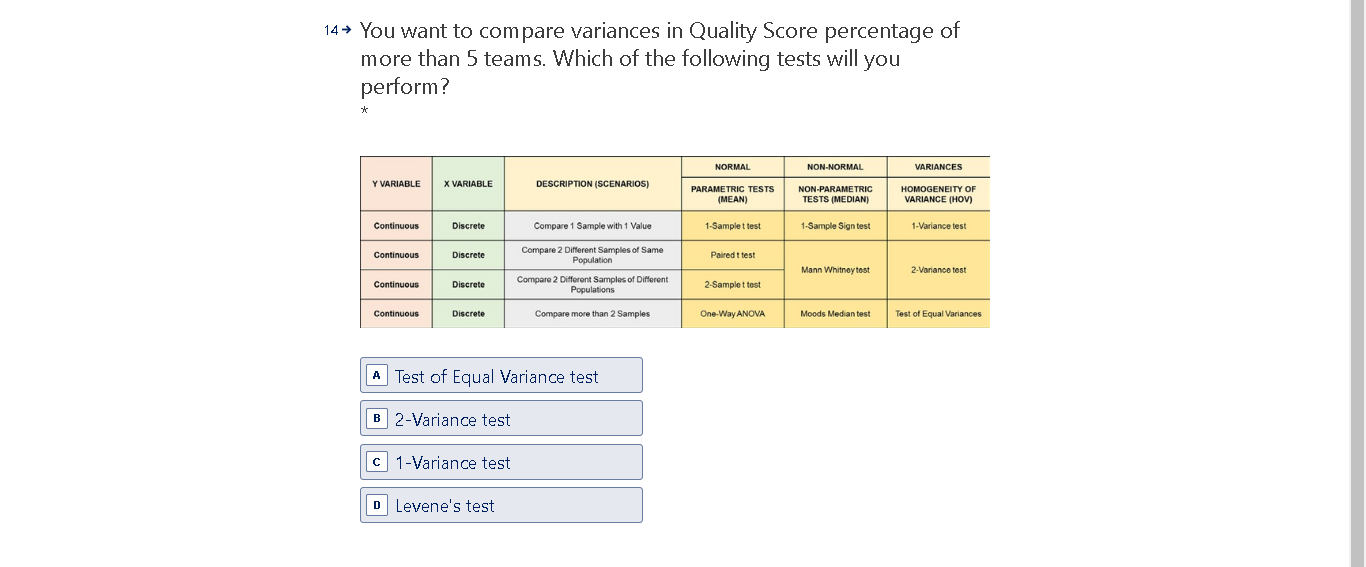# Question Solved1 Answer14+ You want to compare variances in Quality Score percentage of more than 5 teams. Which of the following tests will you perform? ok NORMAL NON-NORMAL VARIANCES Y VARIABLE X VARIABLE DESCRIPTION (SCENARIOS) PARAMETRIC TESTS (MEAN) NON PARAMETRIC TESTS (MEDIAN) HOMOGENEITY OF VARIANCE (HOV) Continuous Discrete Compare 1 Sample with 1 Value 1-Samplet test 1-Sample Sign test 1-Variance test Continuous Discrete Pairedt test Compare 2 Different Samples of Same Population Compare 2 Different Samples of Different Populations Mann Whitneytost 2-Variance test Continuous Discrete 2-Samplet test Continuous Discrete Compare more than 2 Samples One-Way ANOVA Moods Median test Test of Equal Variances A Test of Equal Variance test B 2-Variance test C 1-Variance test D Levene's testUV1BTR The Asker · Probability and StatisticsTranscribed Image Text: 14+ You want to compare variances in Quality Score percentage of more than 5 teams. Which of the following tests will you perform? ok NORMAL NON-NORMAL VARIANCES Y VARIABLE X VARIABLE DESCRIPTION (SCENARIOS) PARAMETRIC TESTS (MEAN) NON PARAMETRIC TESTS (MEDIAN) HOMOGENEITY OF VARIANCE (HOV) Continuous Discrete Compare 1 Sample with 1 Value 1-Samplet test 1-Sample Sign test 1-Variance test Continuous Discrete Pairedt test Compare 2 Different Samples of Same Population Compare 2 Different Samples of Different Populations Mann Whitneytost 2-Variance test Continuous Discrete 2-Samplet test Continuous Discrete Compare more than 2 Samples One-Way ANOVA Moods Median test Test of Equal Variances A Test of Equal Variance test B 2-Variance test C 1-Variance test D Levene's test
More
Transcribed Image Text: 14+ You want to compare variances in Quality Score percentage of more than 5 teams. Which of the following tests will you perform? ok NORMAL NON-NORMAL VARIANCES Y VARIABLE X VARIABLE DESCRIPTION (SCENARIOS) PARAMETRIC TESTS (MEAN) NON PARAMETRIC TESTS (MEDIAN) HOMOGENEITY OF VARIANCE (HOV) Continuous Discrete Compare 1 Sample with 1 Value 1-Samplet test 1-Sample Sign test 1-Variance test Continuous Discrete Pairedt test Compare 2 Different Samples of Same Population Compare 2 Different Samples of Different Populations Mann Whitneytost 2-Variance test Continuous Discrete 2-Samplet test Continuous Discrete Compare more than 2 Samples One-Way ANOVA Moods Median test Test of Equal Variances A Test of Equal Variance test B 2-Variance test C 1-Variance test D Levene's test